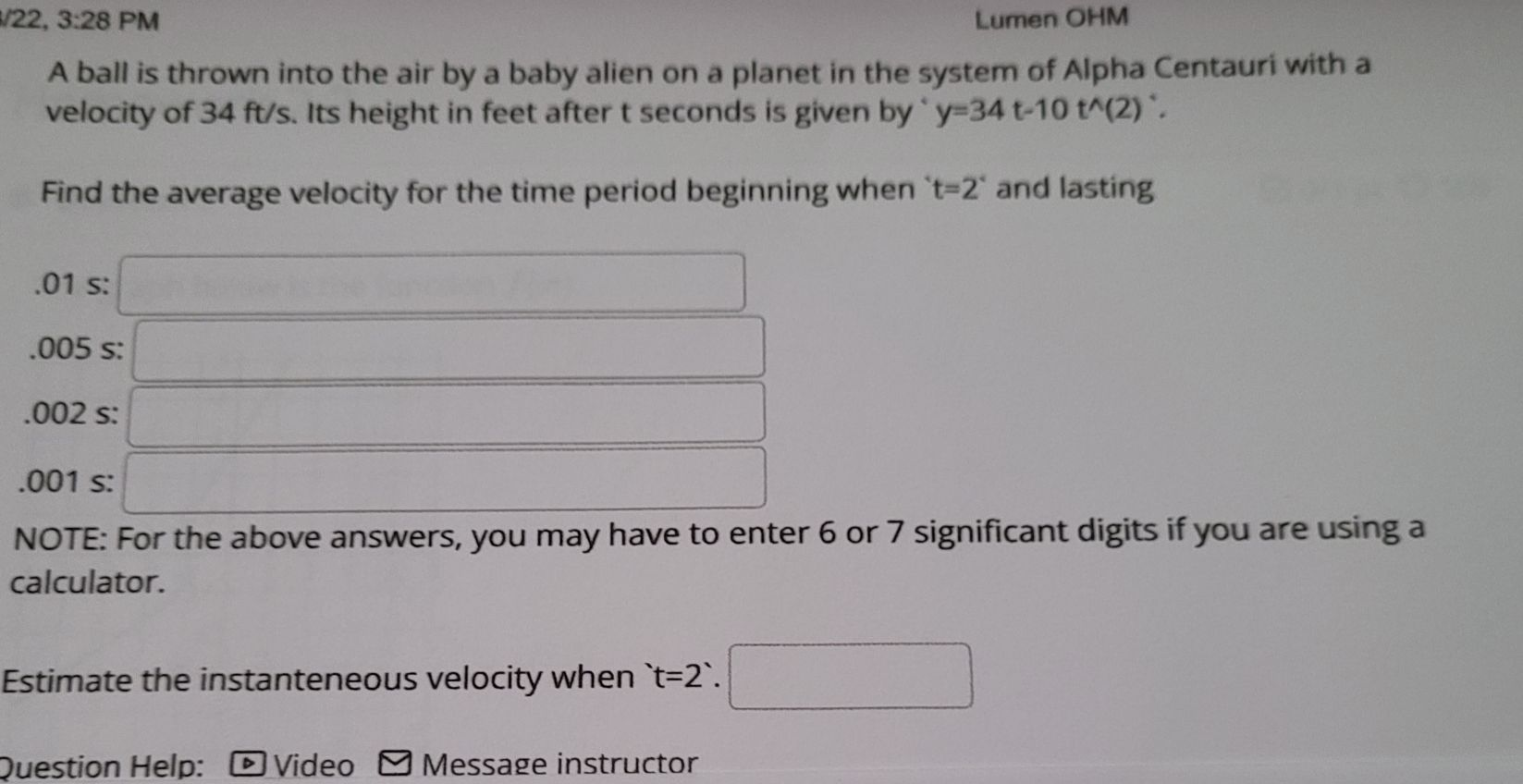### ¿Todavía tienes preguntas de matemáticas?

Pregunte a nuestros tutores expertos
Algebra
PreguntaA ball is thrown into the air by a baby alien on a planet in the system of Alpha Centauri with a velocity of $$34 ft / s$$ . Its height in feet after $$t$$ seconds is given by " $$y = 34 t - 10 t ^ { \wedge } ( 2 ) ^ { \circ }$$ .

Find the average velocity for the time period beginning when $$t = 2 ^ { \circ }$$ and lasting

$$.01 s :$$

$$.005 s :$$

$$.002 s :$$

$$.001 s :$$

NOTE: For the above answers, you may have to enter $$6$$ or $$7$$ significant digits if you are using a calculator.

Estimate the instanteneous velocity when 't=2'.